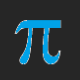# A Comparison of the Areas of Some of the Triangles Formed By Connecting Three Noncollinear Triangle Centers

The five most well-known triangle centers are the centroid (where a triangle’s medians meet), the orthocenter (where the lines containing the altitudes meet), the incenter (where a triangle’s three interior angle bisectors meet), the circumcenter (where the perpendicular bisectors of a triangle’s three sides meet), as well as the center of a triangle’s 9-point circle (see https://en.wikipedia.org/wiki/Nine-point_circle for more information on this circle, and how it is defined). In the diagram below, the constructions for all five of these triangle centers have been performed, for obtuse, scalene triangle ABC.The thick pink line is called the Euler line, and four of the five triangle centers mentioned above — all of them except the incenter — are always located on this line, no matter a triangle’s shape or size. The incenter, however, is only found on the Euler line for isosceles or equilateral triangles, so, for such triangles, all five of these triangle centers are collinear — and, as a consequence, no triangles can be made by connecting any set of three of them. If the triangle is scalene, however, the incenter will leave the Euler line, and these triangles may then be defined (with construction-clutter removed, but for the same triangle ABC as shown in the first diagram):If A, B, and/or C are moved around, the area of triangle ABC changes, as do, of course, the areas of the colored triangles above, of which there are six:  yellow, red, blue, yellow and red together, blue and red together, and all three taken as one triangle. For the original configuration of triangle ABC, you can see those triangle areas on the right side of the image above. On the left side, various ratios are given:

• The triangle which joins the incenter, 9-point circle center, and circumcenter has the same area as the triangle joining the incenter, 9-point circle center, and the orthocenter.
• The triangle joining the incenter, centroid, and orthocenter has twice the area of the triangle joining the incenter, centroid, and circumcenter — and this latter triangle, itself, has twice the area of the triangle joining the incenter, centroid, and 9-point circle center.
• The area of the triangle connecting the incenter, orthocenter, and circumcenter has an area three times as large as the triangle connecting the incenter, centroid, and circumcenter.
• As a consequence of the last two bulleted statements, the area of the triangle connecting the incenter, orthocenter, and circumcenter is six times the area of the triangle connecting the incenter, centroid, and 9-point circle center.

In both diagrams above, the original triangle ABC is scalene and obtuse. If A, B, and/or C are moved around, but the triangle remains scalene (so that the five triangle centers in question remain noncollinear), all six of the colored triangles described above still exist — and the area ratios given in the bulleted statements above remain constant, also. I do not yet have proofs for the constancy of these area ratios, but am confident that it is possible to write them.

If A, B, and C are positioned in such a way that triangle ABC is almost equilateral, the five triangle centers discussed here get very close together — because for a triangle which actually is regular, all five are located in exactly the same spot. Here’s what the almost-regular case looks like:As you can see, the area ratios described above (left side of diagram) remain the same, even as the actual colored-triangle areas (right side) all approach zero. If I complete a proof for the constancy of any or all of these area ratios, I’ll post such proofs in subsequent posts on this blog — or readers are welcome to write their own proofs, and are invited to leave them as comments on this post.

## 2 thoughts on “A Comparison of the Areas of Some of the Triangles Formed By Connecting Three Noncollinear Triangle Centers”

1. This seems much more involved than the last one. 🙂

Liked by 1 person

2.RobertLovesPi on said:

Thank you!

Like